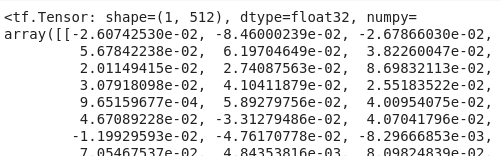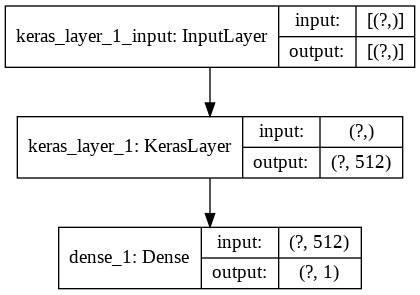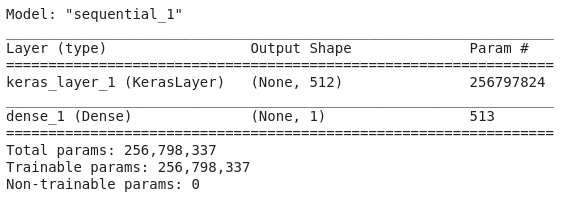# Transfer Learning in NLP with Tensorflow Hub and Keras

Tensorflow 2.0 introduced Keras as the default high-level API to build models. Combined with pretrained models from Tensorflow Hub, it provides a dead-simple way for transfer learning in NLP to create good models out of the box.To illustrate the process, let’s take an example of classifying if the title of an article is clickbait or not.

## Data Preparation

We will use the dataset from the paper ‘Stop Clickbait: Detecting and Preventing Clickbaits in Online News Media’ available here.

import pandas as pd


The dataset consists of page titles and labels. The label is 1 if the title is clickbait.Let’s split the data into 70% training data and 30% validation data.

from sklearn.model_selection import train_test_split

x_train, x_test, y_train, y_test = train_test_split(df['title'],
df['label'],
test_size=0.3,
stratify=df['label'],
random_state=42)


## Model Architecture

Now, we install tensorflow and tensorflow-hub using pip.

pip install tensorflow-hub
pip install tensorflow==2.1.0


To use text data as features for models, we need to convert it into a numeric form. Tensorflow Hub provides various modules for converting the sentences into embeddings such as BERT, NNLM and Wikiwords.

Universal Sentence Encoder is one of the popular module for generating sentence embeddings. It gives back a 512 fixed-size vector for the text. Below is an example of how we can use tensorflow hub to capture embeddings for the sentence “Hello World”.import tensorflow_hub as hub

encoder(['Hello World'])In Tensorflow 2.0, using these embeddings in our models is a piece of cake thanks to the new hub.KerasLayer module. Let’s design a tf.keras model for the binary classification task of clickbait detection.

First import the required libraries.

import tensorflow as tf
import tensorflow_hub as hub


Then, we create a sequential model that will encapsulate our layers.

model = tf.keras.models.Sequential()


The first layer will be a hub.KerasLayer from where we can loading models available at tfhub.dev. We will be loading Universal Sentence Encoder.

model.add(hub.KerasLayer('https://tfhub.dev/google/universal-sentence-encoder/4',
input_shape=[],
dtype=tf.string,
trainable=True))


Here are what the different parameters used mean:

• /4: It denotes the variant of Universal Sentence Encoder on hub. We’re using the Deep Averaging Network (DAN) variant. We also have Transformer architecture and other variants.
• input_shape=[]: Since our data has no features but the text itself, so there feature dimension is empty.
• dtype=tf.string: Since we’ll be passing raw text itself to the model
• trainable=True: Denotes whether we want to finetune USE or not. We set it to True, the embeddings present in USE are finetuned based on our downstream task.

Next, we add a Dense layer with single node to output probability of clickbait between 0 and 1.

model.add(tf.keras.layers.Dense(1, activation='sigmoid'))


In summary, we have a model that takes text data, projects it into 512-dimension embedding and passed that through a feedforward neural network with sigmoid activation to give a clickbait probability.Alternatively, we can implement the exact above architecture using the tf.keras functional API as well.

x = tf.keras.layers.Input(shape=[], dtype=tf.string)
trainable=True)(x)
z = tf.keras.layers.Dense(1, activation='sigmoid')(y)
model = tf.keras.models.Model(x, z)


The output of the model summary is

model.summary()The number of trainable parameters is 256,798,337 because we’re finetuning Universal Sentence Encoder.

## Training the model

Since we’re performing a binary classification task, we use a binary cross entropy loss along with ADAM optimizer and accuracy as the metric.

model.compile(optimizer='adam',
loss='binary_crossentropy',
metrics=['accuracy'])


Now, let’s train the model for

model.fit(x_train,
y_train,
epochs=2,
validation_data=(x_test, y_test))


We reach a training accuracy of 99.62% and validation accuracy of 98.46% with only 2 epochs.

## Inference

Let’s test the model on a few examples.

# Clickbait
>> model.predict(["21 Pictures That Will Make You Feel Like You're 99 Years Old"])
array([[0.9997924]], dtype=float32)

# Not Clickbait
>> model.predict(['Google announces TensorFlow 2.0'])
array([[0.00022611]], dtype=float32)


## Conclusion

Thus, with a combination of Tensorflow Hub and tf.keras, we can leverage transfer learning easily and build high-performance models for any of our downstream tasks.

## Data Credits

Abhijnan Chakraborty, Bhargavi Paranjape, Sourya Kakarla, and Niloy Ganguly. "Stop Clickbait: Detecting and Preventing Clickbaits in Online News Media”. In Proceedings of the 2016 IEEE/ACM International Conference on Advances in Social Networks Analysis and Mining (ASONAM), San Fransisco, US, August 2016

Categories:

Updated: